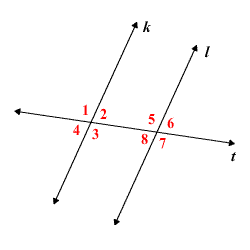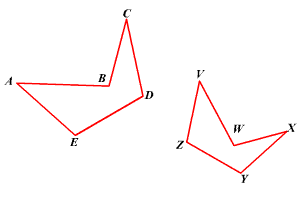# Corresponding Angles

The term corresponding angles is often used when two lines are cut by a third line, a transversal .In the figure above, line $t$ is a transversal cutting lines $k$ and $l$ , and there are four pairs of corresponding angles:

$\angle 1$ and $\angle 5$

$\angle 2$ and $\angle 6$

$\angle 3$ and $\angle 7$

$\angle 4$ and $\angle 8$

The Corresponding Angles Postulate states that if $k$ and $l$ are parallel , then the pairs of corresponding angles are congruent . The converse of this theorem is also true.

The term corresponding angles is also sometimes used when making statements about similar or congruent polygons . For example, in the figure below featuring two similar pentagons, $\angle A$ and $\angle V$ are corresponding angles.